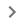# TO_PERCENT

Converts a provided number to a percentage.

### Sample Usage

`TO_PERCENT(A2)`

`TO_PERCENT(0.40826)`

### Syntax

`TO_PERCENT(value)`

• `value` - The argument or reference to a cell to be converted to a percentage.

• If `value` is a number or a reference to a cell containing a numeric value, `TO_PERCENT` returns `value` converted to a percentage, with the standard interpretation that 1 = 100%.

• If `value` is not a number or a reference to a cell containing a numeric value, `TO_PERCENT` returns `value` without modification.

### Notes

• Because dates and percentages are both numbers, `TO_PERCENT` will convert dates to percentages successfully. However, these conversions are not typically meaningful.

• `TO_PERCENT` is equivalent to clicking FormatNumberPercent from the menu bar.

`UNARY_PERCENT`: Returns a value interpreted as a percentage; that is, `UNARY_PERCENT(100)` equals `1`.

`TO_TEXT`: Converts a provided numeric value to a text value.

`TO_PURE_NUMBER`: Converts a provided date/time, percentage, currency or other formatted numeric value to a pure number without formatting.

`TO_DOLLARS`: Converts a provided number to a dollar value.

`TO_DATE`: Converts a provided number to a date.

`N`: Returns the argument provided as a number.